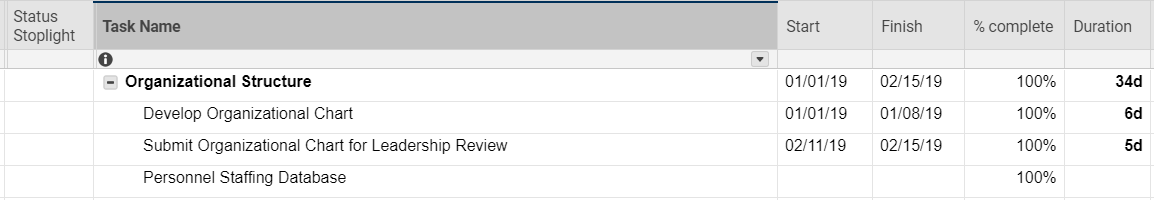# automate RYG balls

I'm a smartsheet newbie and i'm ultimately trying to build a formula that automates task progress based on the following:

If % Complete = 100, return Blue

If it’s not scheduled to start yet, return Clear

If it’s past the scheduled finish date, return Red

If % complete >= (today date-start date)/task duration, return Green

Otherwise, return Yellow.

After researching if statements i wanted to at least start with the first simple if statement to return blue ball if task is 100% complete. Below is what i used for the formula but nothing returns in the cell.

=IF([% complete]1 = 100, "Blue")

attached screenshotTags:

• You're very close. In formulas, % complete is a range of 0-1, so it should be:

=IF([% complete]1 = 1, "Blue")

If you were looking for over 50% it would look like this:

=IF([% complete]1 > .5, "Blue")

Let me know if you need help with the rest of the formula.

• Thank you!!  I’m obsessed w making this formula work and figure if i chunk out the pieces then I can just tie it all in after I test each piece. This was the easiest piece.  Ok. Well I’m onto the next piece. Hope you’re online for my next question!  Really appreciate the quick response.

• I figured it out, here's the breakdown so that my RYG status indicator is automated based on the below, and you only have to put in your manual % completes.  Not bad as i've never used smartsheet and i am basic excel user, never used MS project• If % Complete = 100, return Blue
• =IF([% complete]1 = 1, "Blue")
• If it’s not scheduled to start yet, and % complete is blank, return Clear
• =IF(AND(TODAY() < [Start date]7, ISBLANK([% Complete]7)), "")
• If it’s past the scheduled end date and % complete is less than 100%, return Red
• =IF(AND(TODAY() > [End date]2, [% Complete]2 < 1), "Red")
• If % complete < the prorated expected completion (If the percent complete is less than the number of days since the task was scheduled to begin, divided by the task duration, it will be Yellow; otherwise, it will be Green.
• =IF([% Complete]3 < (TODAY() - [Start date]3) / Duration3, "Yellow", "Green")
• All of the above combined
• =IF([% Complete]10 = 1, "Blue", IF(AND(TODAY() < [Start date]10, ISBLANK([% Complete]10)), "", IF(TODAY() > [End date]10, "Red", IF([% Complete]10 < (TODAY() - [Start date]10) / Duration10, "Yellow", "Green"))))
• Nicely done. Those nested IF statements can get tricky.

## Help Article Resources

Want to practice working with formulas directly in Smartsheet?

Check out the Formula Handbook template!